# 还在划水？这个 SQL 你能写出来吗？有一张表b字段包括：用户id，年，月，请查询在2020年每个月都有记录的用户id?

select user_id from b where year=2020 group by user_id having count(month)=12;

CREATE TABLE b (  user_id int(11) NOT NULL,  month int(10) DEFAULT NULL,  year int(10) DEFAULT NULL) ENGINE=InnoDB DEFAULT CHARSET=latin1;

insert into b values(1,1,2020),(1,2,2020),(1,3,2020),(1,4,2020),(1,5,2020),(1,6,2020),(1,7,2020),(1,8,2020),(1,9,2020),(1,10,2020),(1,11,2020),(1,12,2020),(1,1,2020);insert into b values(2,1,2020),(2,2,2020),(2,3,2020),(2,4,2020),(2,5,2020),(2,6,2020),(2,7,2020),(2,8,2020),(2,9,2020),(2,10,2020),(2,11,2020),(2,12,2020);insert into b values(6,1,2020),(6,2,2020),(6,3,2020),(6,4,2020),(6,5,2020),(6,6,2020),(6,7,2020),(6,8,2020),(6,9,2020),(6,10,2020),(6,11,2020),(6,12,2020);insert into b values(10,1,2020),(10,2,2020),(10,3,2020),(10,4,2020),(10,5,2020),(10,6,2020),(10,7,2020),(10,8,2020),(10,9,2020),(10,10,2020),(10,11,2020),(10,12,2020);insert into b values(25,1,2020),(25,2,2020),(25,3,2020),(25,4,2020),(25,5,2020),(25,6,2020),(25,7,2020),(25,8,2020),(25,9,2020),(25,10,2020),(25,11,2020),(25,12,2020);insert into b values(66,1,2020),(66,2,2020),(66,6,2020),(66,4,2020),(66,5,2020),(66,6,2020),(66,7,2020),(66,8,2020),(66,9,2020),(66,10,2020),(66,11,2020),(66,12,2020);insert into b values(7,1,2020),(7,2,2020),(7,4,2020),(7,5,2020),(7,7,2020),(7,9,2020),(7,10,2020),(7,11,2020);insert into b values(12,1,2020),(12,4,2020),(12,5,2020),(12,7,2020),(12,9,2020),(12,10,2020),(12,11,2020);insert into b values(12,1,2019),(12,4,2019),(12,5,2019),(12,7,2019),(12,9,2019),(12,10,2019),(12,11,2019);

select DISTINCT month,user_id from b where year=2020;

select user_id,DISTINCT month from b where year=2020;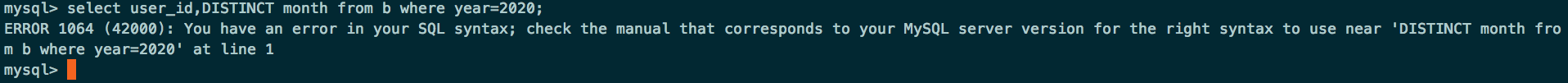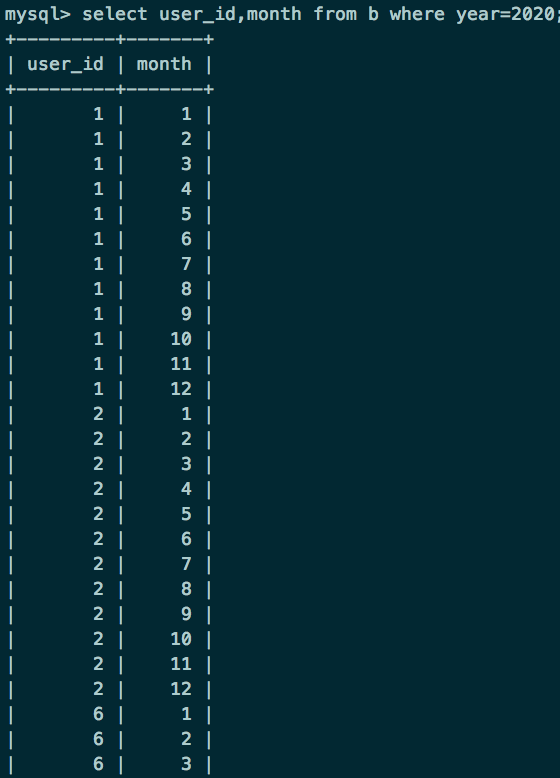select user_id from 表1 as c group by c.user_id having count(month)=12;

OK，那到这里基本就出来了，表1是个子查询

select user_id from (select DISTINCT month,user_id from b where year=2020) as c group by c.user_id having count(month)=12;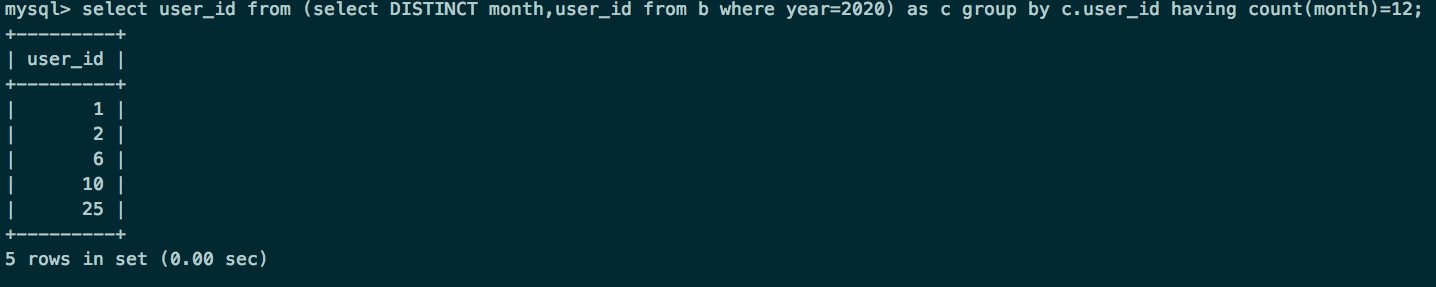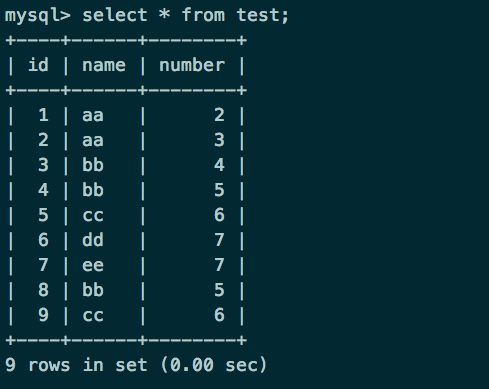SELECT name FROM test GROUP BY name;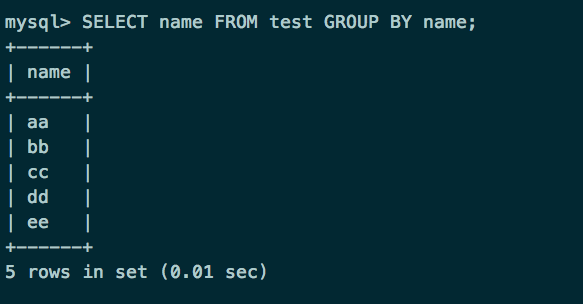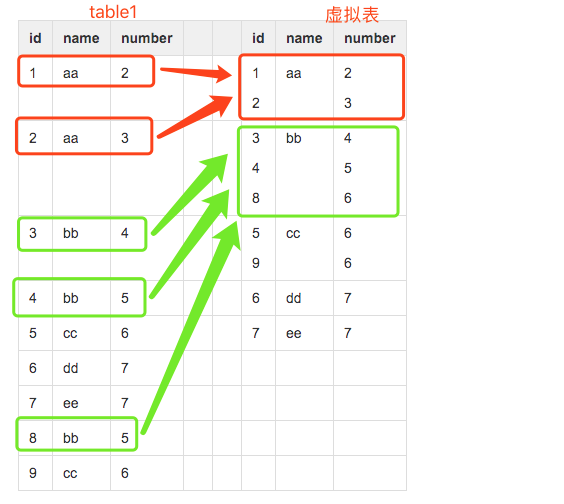• 再看name列，每个单元格只有一个数据，所以我们select name的话，就没有问题了。为什么name列每个单元格只有一个值呢？因为我们就是用name列来group by的

• 那么对于id和number里面的单元格有多个数据的情况怎么办呢？答案就是用聚合函数，聚合函数就用来输入多个数据，输出一个数据的。如cout(id),sum(number)，而每个聚合函数的输入就是每一个多数据的单元格

• 例如我们执行select name,sum(number) from test group by name，那么sum就对虚拟表的number列的每个单元格进行sum操作，例如对name为aa的那一行的number列执行sum操作，即2+3，返回5，最后执行结果如下：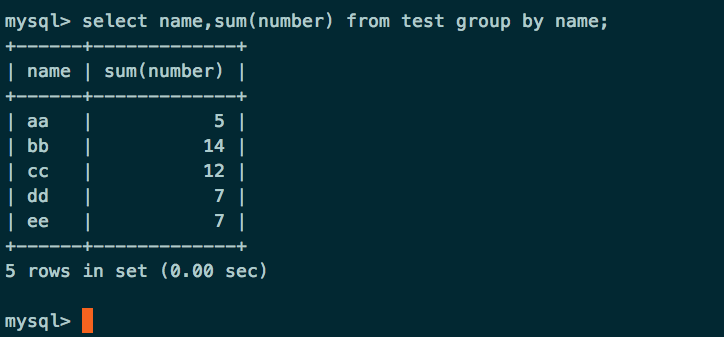group by执行原理参考：

https://blog.csdn.net/qq403580298/article/details/90756352## 评论